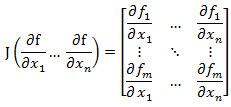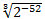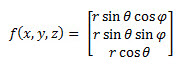# SQL Server JACOBIAN function

JACOBIAN
Updated: 16 April 2018

Use the scalar function JACOBIAN to numerically compute the Jacobian matrix. The Jacobian matrix is the matrix of all first-order partial derivatives of a vector-valued function.Syntax
SELECT [wct].[JACOBIAN] (
<@Func, nvarchar(max),>
,<@VarNames, nvarchar(4000),>
,<@X, nvarchar(4000),>
,<@H, float,>)
Arguments
 Input Name Description @Func The function to be evaluated, as a string. The function must be in the form of a SELECT statement. @VarName The name of the variable @X The point where the Jacobian is calculated @H Step size
Return Type
[nvarchar](max)
Remarks
• If @Func returns a NULL then NULL Is returned.
• If @Func is not a valid SELECT statement then NULL is returned.
• If no solution is found then NULL is returned.
• If @X is NULL then @X = ''.
• If @H is NULL then @H = 0.
• If @H <= 0 then @H =• Available in XLeratorDB / math 2008 only
Examples
Example #1

Calculate the Jacobian for the functionAt the point (2.5,7/16*π,63/128* π)

Since the result is returned as a string, we will use the MATRIX function to unpack the results into a matrix format.

DECLARE @r as float = 2.5
DECLARE @theta as float = 7*PI()/16
DECLARE @phi as float = 63*PI()/128
DECLARE @func as varchar(max) = '
SELECT
@r*SIN(@theta)*COS(@Phi) as x
,@r*SIN(@theta)*SIN(@Phi) as y
,@r*COS(@theta) as z'
DECLARE @varnames as varchar(max) = '@r,@theta,@phi'
DECLARE @X as varchar(max) = wct.MATRIX2STRING_q('SELECT 2.5, 7*PI()/16,63*PI()/128')
DECLARE @h as float = wct.POWER(2,-20)

SELECT
*
FROM
wct.MATRIX(
wct.JACOBIAN(
@func
,@varnames
,@X
,@h
)
)

This produces the following result.Copyright 2008-2021 Westclintech LLC         Privacy Policy        Terms of Service